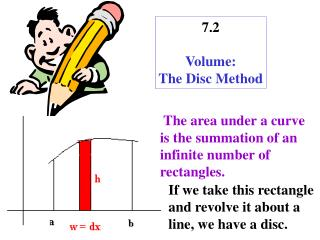DownloadDownload Presentation7.2 Volume: The Disc Method

# 7.2 Volume: The Disc Method

Download Presentation## 7.2 Volume: The Disc Method

- - - - - - - - - - - - - - - - - - - - - - - - - - - E N D - - - - - - - - - - - - - - - - - - - - - - - - - - -
##### Presentation Transcript

1. 7.2 Volume: The Disc Method The area under a curve is the summation of an infinite number of rectangles. If we take this rectangle and revolve it about a line, we have a disc.

2. This disc is nothing more than a cylinder on it’s side. r The volume of a cylinder is: Disc If we sum up an infinite number of cylinders, we have the new integration formula: r = h & w = dx This equation is for a horizontal axis of revolution.

3. Vertical axis of revolution

4. Find the radius for y = x2, y = 0, and x = 2 about the line x = 2 about the line x = 3 4 4 2 x r = 2 - x x = 3